Courses
Courses for Kids
Free study material
Free LIVE classes
More

# Multiplicative Identity Property (Number Multiplied by 1)LIVE
Join Vedantu’s FREE Mastercalss

## Overview of Multiplication

Have you ever heard about the answer obtained when any number is multiplied by 1? When any number is multiplied by 1 the product is always the number itself. In the below-discussed article, children would gain knowledge about the steps involved in solving multiplication problems, which is considered one of the basic and most widely used mathematical and arithmetical operations. Generally, it is represented using the symbol '×' or '*' in between the two numbers. Now, let us start with our topic here:

## What is Multiplication?

Multiplication is a process of calculating the product of two quantities or numbers by multiplying them together. '×' is used to depict the product of two quantities. The number multiplied by the multiplier is called the multiplicand and the number by which the multiplicand is multiplied is called the multiplier. It can also be stated as a way of adding a number repeatedly to acquire their product.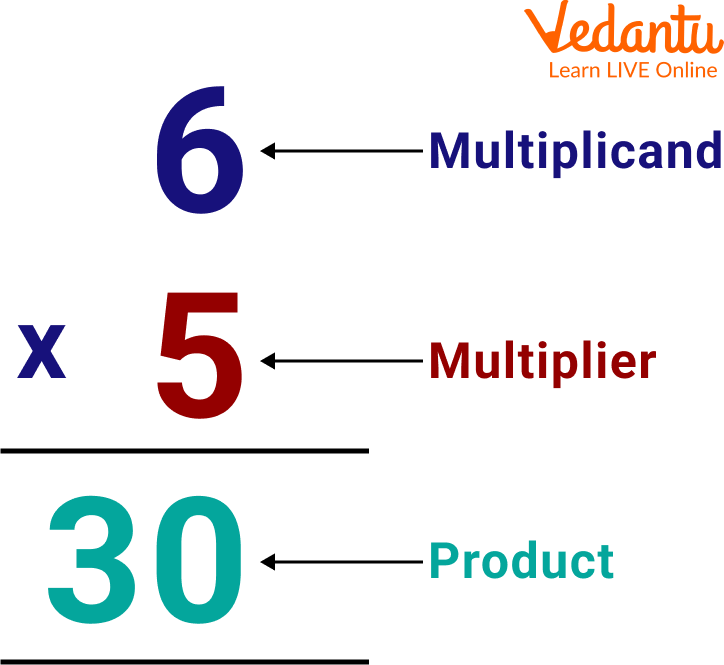Showing Terms Used in the Multiplication of Two Numbers

## Steps Involved in Solving Multiplication Queries

The steps required to follow in the multiplication of two numbers are given below:

• Write the given multiplicand and multiplier in the column form by taking into consideration their place values

• Then put the multiplication sign (×) preceding the multiplier

• Start the multiplication from the right-hand side of the multiplier and move to the left side.

• Count the number of digits in multiplier quantity and place the zeroes 1 less than the number of digits in the multiplier from the right side

• Finally, add up all the products respective to each digit of the multiplier.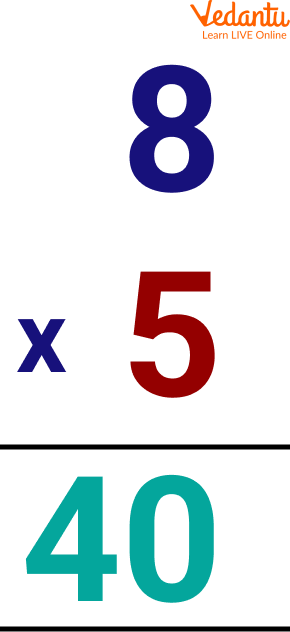Multiplication of 8 and 5

## Multiplicative Identity Property

Multiplicative identity property states that "Any number multiplied by 1 is the number itself". It means that when any number is multiplied by 1 the answer is always the number. It serves as a user identity in solving various problems. 1 is the identity of itself i.e. 1 × 1 = 1. It is also called the Identity Property of Multiplication because of the reason, here the identity of a number remains unaltered.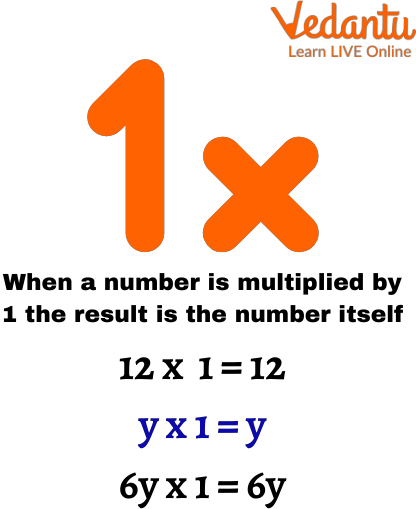Showing Identity Property of Multiplication

## Solved Examples

Q 1. 12 × 1

Ans: Steps to be followed to calculate the product using Multiplicative Identity

• Align the given numbers in columns or rows to calculate the product

• Multiplying 1 with the given number and noting the result

Thus, we can conclude that when any number is multiplied by 1 the answer is the number itself.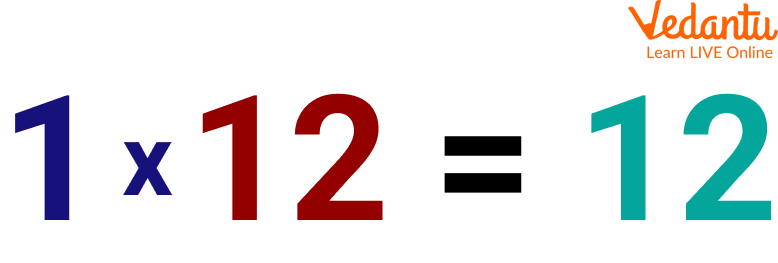Showing Any Number Multiplied by 1

Q 2. 65 × 31

Ans: Steps to calculate the result of this statement are given below:

• Write the given multiplier and multiplicand in columns format

• Now, using multiplicative identity, any number multiplied by 1 is the number, for multiplying 65 and 1

• Calculate the number of digits of the multiplier and put zeroes one less than the calculated number of digits

• Further, multiplying the tens digit of the multiplier with the multiplicand

• Adding the two obtained partial products i.e. 65 + 1950 = 2015

Hence, the required result of the multiplication of 65 × 31 is 2015.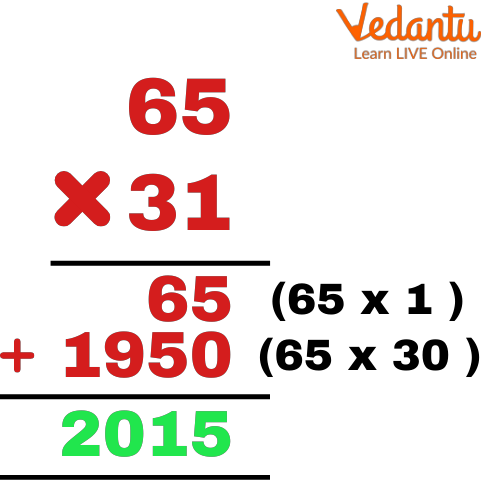Showing the Multiplication of Two Digit Number Whose One Digit is 1

## Practice Problems

Some practice problems based on the concept, any number multiplied by 1 is the number itself are given below. These should be solved by the children on their own for a better understanding of the concepts.

Q 1. 59 × 1

Ans: 59

Q 2. 84 × 1

Ans: 84

Q 3. 1 × 18

Ans: 18

Q 4. 34 × 1

Ans: 34

Q 5. 1 × 32

Ans: 32

## Summary

To wrap up here with the topic of number multiplication. The main motive of this article is to impart knowledge of multiplication by covering every topic including, what is multiplication, the steps involved in solving mathematics problems, whether any number multiplied by 1 is the number, etc. It is formed using the solved examples and images which makes learning interesting and exciting. Hoping the writing helped you in capturing the concept of multiplication and you enjoyed reading it. Feel comfortable to ask about your problems.

Last updated date: 25th Sep 2023
Total views: 93.3k
Views today: 2.93k

## FAQs on Multiplicative Identity Property (Number Multiplied by 1)

1. What is the general use of multiplication?

The most general use of multiplication is when we need to calculate a large number of things for a given small amount. Multiplication is nothing but a less time taking process of repetitive addition. So it takes less time to calculate the sum. The multiplication rule is also used to calculate the probability of occurrence of two events.

2. Can the multiplier and multiplicand be the same?

Yes, a multiplier and multiplicand can be the same in a multiplication problem. There is no such rule in maths that these both should be different. When a number is multiplied by its own, the result is always the square of the given number. For example 2 × 2 = 2² = 4, 13 × 13 = 13² = 169, etc.

3. What happens when one is multiplied by zero?

When 1 is multiplied by 0, the answer is always zero. However, we know that when any number is multiplied by 1 the product is the number itself, similarly we know that any number multiplied by zero results in zero.